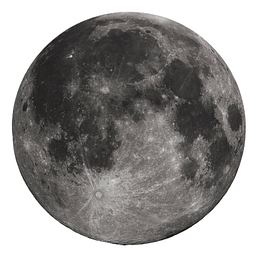# How to Color Your Pandas Dataframe

`import numpy as npimport pandas as pddf = pd.DataFrame(np.random.randint(1000, 10000, size=(5, 5)), columns=list("01234"))`

# Exploratory Data Analysis Made Easy: Using Sweetviz

• A general overview of the dataset
• Categorical associations
• Numerical associations
• Variable properties
• Most frequent, smallest, and largest values for numerical features

# Hyperparameter Tuning in Python: Machine Learning Techniques